## 11. Euler's Gammageneralized binomial coefficientsStirling's approximation to n!

### 1. The Euler's Γ function

The factorial n! of a natural number is recursively defined as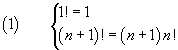L. Euler found that it is possible to define a function of a real (or complex) variable Γ(x) ('Gamma of x') with the same recursive property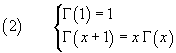If we apply such function to an argument x+1 coincident with a natural number, we have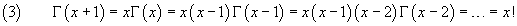So Γ, for natural arguments, produces values identical to those of the factorial

Euler was able to express the function Γ in this way: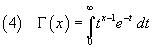In fact the definition (4) gives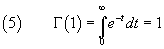and also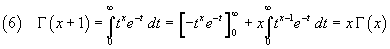In particular, if x coincides with the natural number n, we can write the following equalities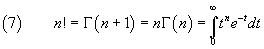Using the second one of the equalities (2), given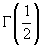, we can calculate the values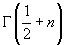for each natural number n ≥ 1.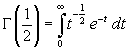With t=z2, we have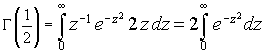The last integral is a well known gaussian integral and has value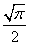. Therefore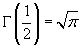The values of Γ for subsequent increments by 1 of the argument can be obtained recursively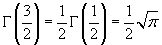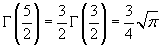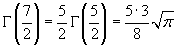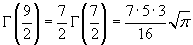In general, for n ≥ 0,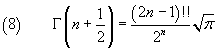In the equality (8) the double question mark represents the double factorial of the natural number before it, that is, if the number is odd, the product of the number itself by all the preceding odd numbers, and, if the number is even, the product of the number itself by all the preceding even numbers. Moreover we assume -1!! = 0!! = 1

The equalities (7) e (8) allow to extend the definition of the factorial from the set of the natural numbers to the set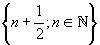in the following way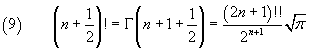In particular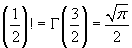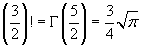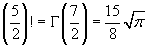From the second one of the equalities (2) we have alsotherefore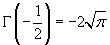and recursively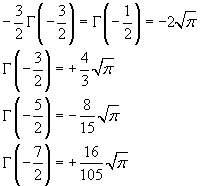In general, for n ≥ 0,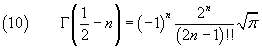We can therefore define the values of the factorials also on the set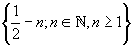in the following way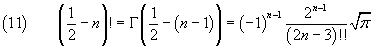In particular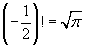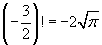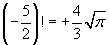The following Javascript application allows you to approximate the values of Γ(x) for real or complex arguments. If your browser does not allow the `iframe` tag, you can directly open its page.
In the input field you can use the decimal digits, the constants P for π, E for the exponential notations and I for the imaginary unit.

### 2. Generalized double factorials

The definition of the double factorial of a natural number, in a similar way as for the factorial, implies the following recursive relation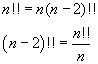if we use these relations for numbers less than 1, we obtain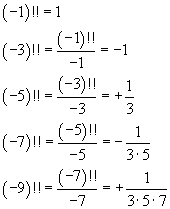In general, for n ≥ 1,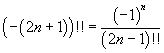Therefore it is possible to calculate the double factorial of a negative integer if it is odd. But this calculation is not possible for a negative integer if it is even because the calculation of (-2)!! implies the division by 0.

### 3. Generalized binomial coefficients

The equality (11) allows us to calculate binomial coefficient like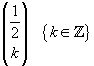.

In fact, if we express binomial coefficients in terms of factorials, we have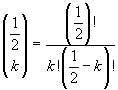If k=0, we have immediately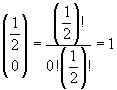and, for k≥1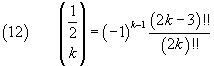In particular: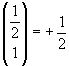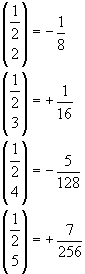If we now compare this sequence with the sequence of the coefficients of the powers of the variable x in the Maclaurin series expansion of the square root of (1-x) (for x real and ≤1)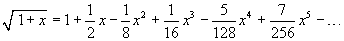we see that these series coincide. Therefore we can write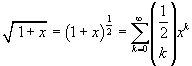This identity allows us to apply something like the binomial theorem also when the exponent of the binomial isn't a natural number.

But we can do the same if, instead of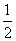, the first term of the binomial coefficient is any real number r. In fact, given G = Γ(r), we have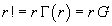and also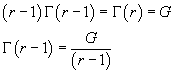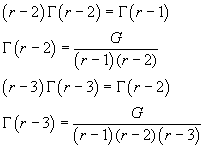In general, for n natural ≥ 1,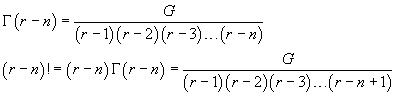Finally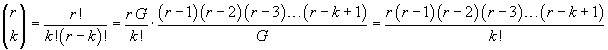and, more synthetically,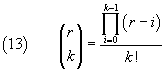Examples.

• Negative integers.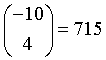• Rational numbers.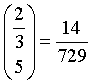• Real numbers.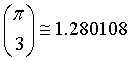• Complex numbers.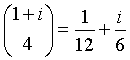The equation (13) allows us to obtain the series expansion of many functions avoiding the laborious derivations needed by the method of Maclaurin.

Examples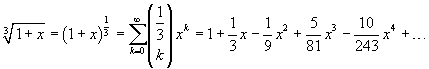WolframAlpha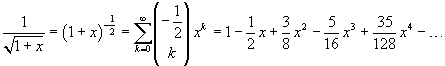WolframAlpha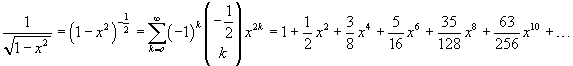WolframAlpha

The following Javascript application allows you to approximate the values of the binomial coefficients for real or complex arguments. If your browser does not allow the `iframe` tag, you can directly open its page.
In the input field you can use the decimal digits, the constants P for π, E for the exponential notations and I for the imaginary unit.

### 4. Stirling's approximation to big factorials

If in the integral in the identity (7) we let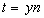we obtain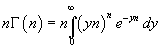that is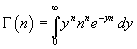If we now multiply and divide the integrand function by en and extract from the integral the factors that does not depend on the integration variable y, we have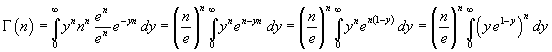The derivative of the integrand function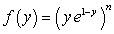with respect to y is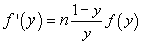therefore f(y) has an absolute maximum for y=1. In order to approximate the integral, we may express it as a function of w = y-1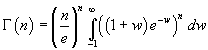and then approximate the integrand function f(w) in the following way (see MathWorld):

• we calculate the logarithm of the function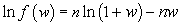• if n is large enough, the function approaches quickly to 0 on the left and on the right of the maximizing argument, so we can assume the absolute value of w near to 0; for w such that |w|<1 we can expand the logarithm using the Mercator's series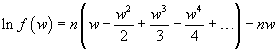and therefore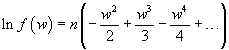If we truncate the expansion at the first order term, we have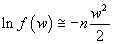that is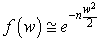Now we have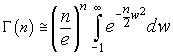• Since by hypothesis the function f(w) goes quickly to 0 in a neighbourhood of 1, the value of this integral does not change appreciably if we extend the limits from -∞ to +∞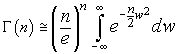The integral in the right side is a Gaussian integral and has value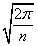; therefore we have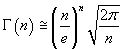and finally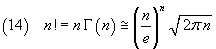The formula (14) is the Stirling's approximation to n! and is very useful in several probabilistic and statistical calculations.

For example:
for 10! instead of the correct value 3628800 it gives 3598697; the error is 8 ‰;
for 100! the error is 0.8 ‰;
for 1000! the error is 0.08 ‰.

It is often useful to calculate the logarithm of the factorial. From (15) we have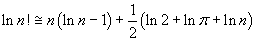For large n the last term is negligible and we can simply write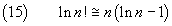The following Javascript application allows you to compare the exact value of the factorial of a natural number with that obtained with the Stirling's approximation.
If your browser does not allow the `iframe` tag, you can directly open its page.